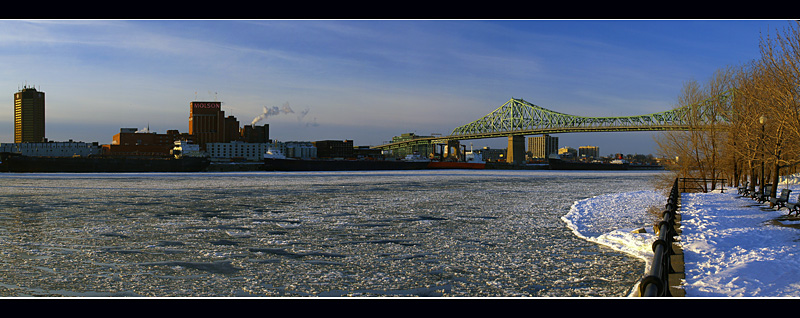Montreal St.-Lawrence River, great Canadian river

Numerical Computation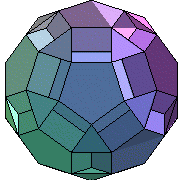The following presents some numerical results on the phase transition problem. For a 2x2 system of conservation laws with artificial viscosity like van der Waals's fluid (or viscoelastic dynamics), the pressure (or the strain function) is non-monotone so that the system with zero viscosity is mixed. Such a problem exhibits three phases: water, water-vapor and vapor phase (or soft material, mixture material and hard material in the case of viscoelasticity), mathematically, corresponding to hyperbolic region, elliptic region and hyperbolic region. Recently, we prove theoretically and numerically that:

1). if the mean value of the initial integral is in the hyperbolic region (water phase, or vapor phase), no matter how small the artificial viscosity is, the global solution uniquely exists and converges to the mean value of the initial integral. In particular, after a short initial oscillation, the solution still keeps in the same hyperbolic region, and no phase transition occurs. See Figure 1.1

2). if the mean value of the initial integral is in the elliptic region (water-vapor phase), and the artificial viscosity is big, the global solution uniquely exists and converges to the mean value of the initial integral. In particular, after a short initial oscillation, the solution still keeps in the same elliptic region, and no phase transition occurs. See Figure 2.1

3). if the mean value of the initial integral is in the elliptic region (water-vapor phase), and the artificial viscosity is small, the global solution oscillates in all time across three phases. The phase transitions occur. See Figure 3.1 for 3-D case, and Figure 3.2, Figure 3.3, Figure 3.4, Figure 3.5 for 2-D case at different times.

Numerical Simulations (click the following small pictures for large)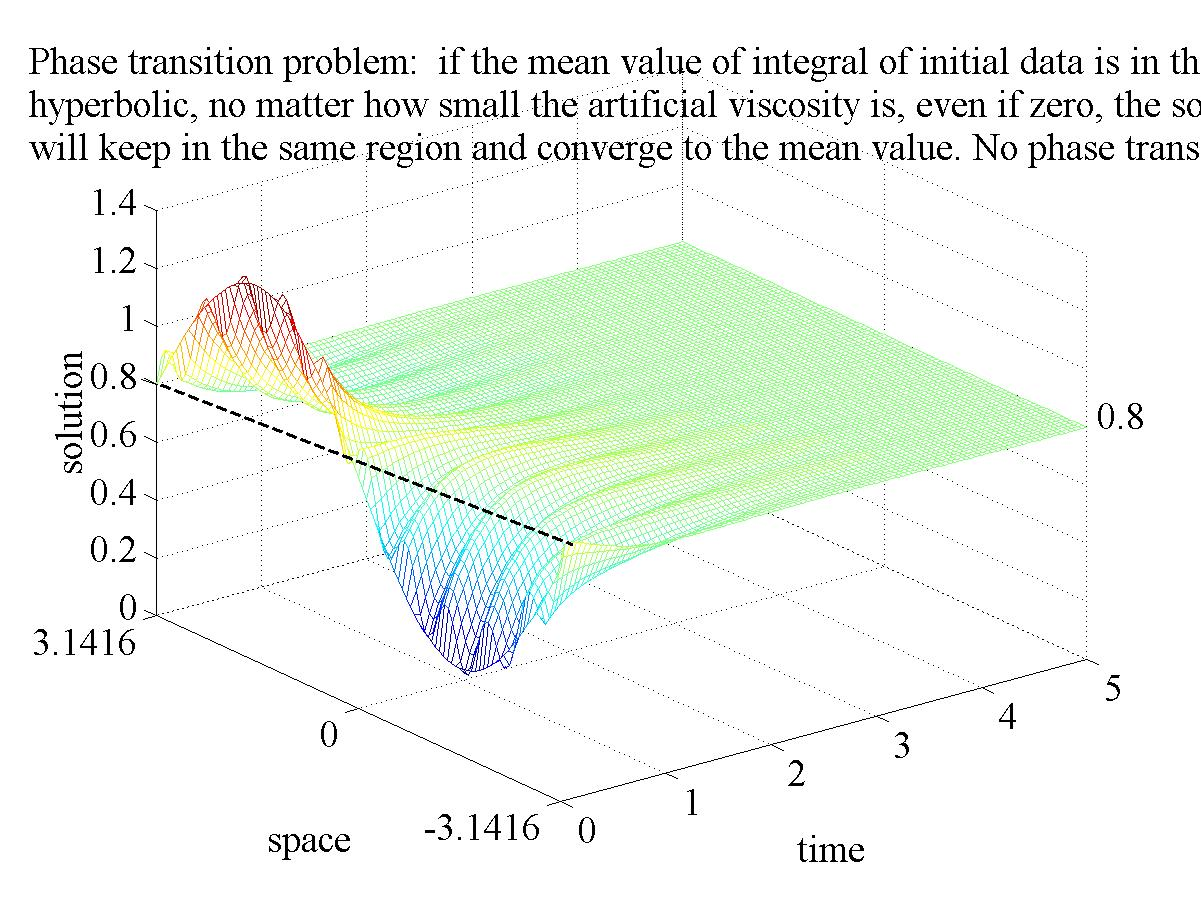Figure 1.1,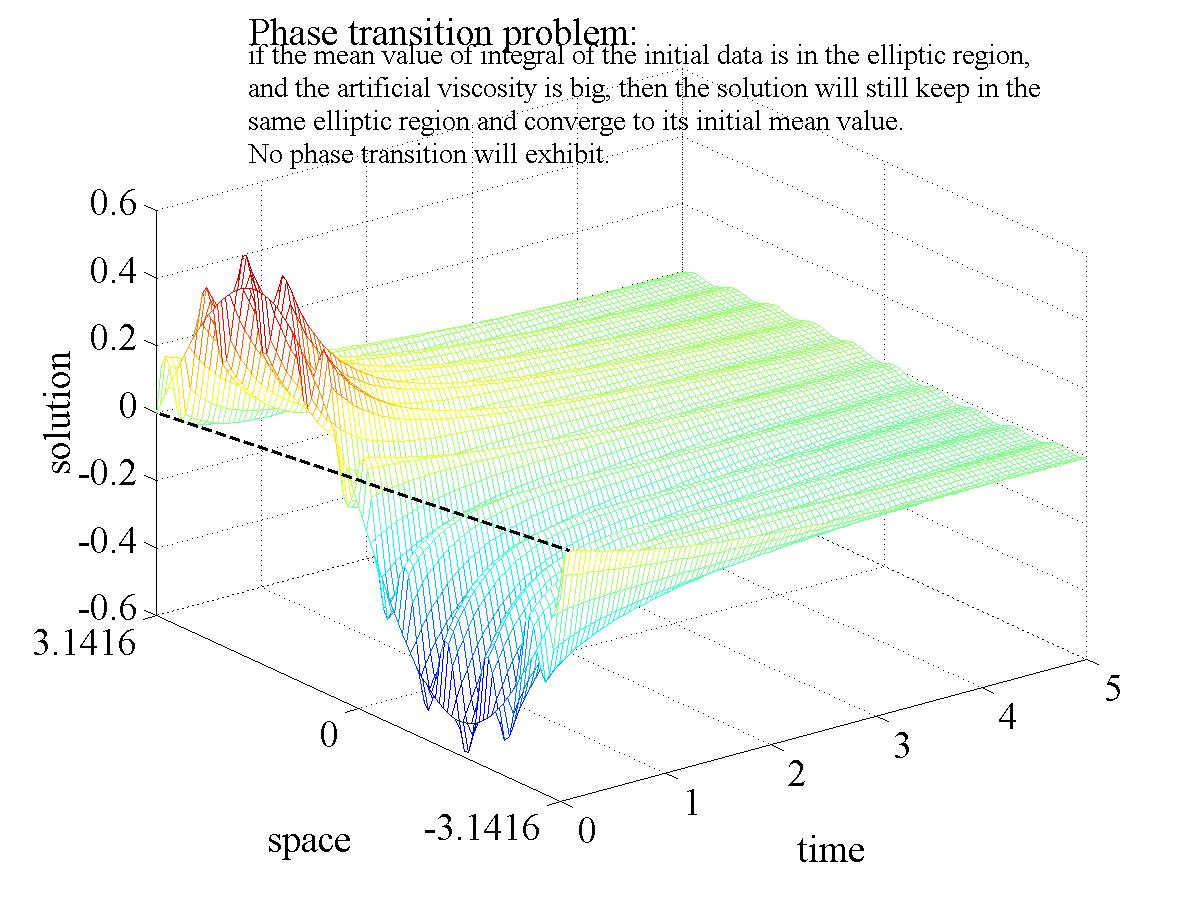Figure 2.1,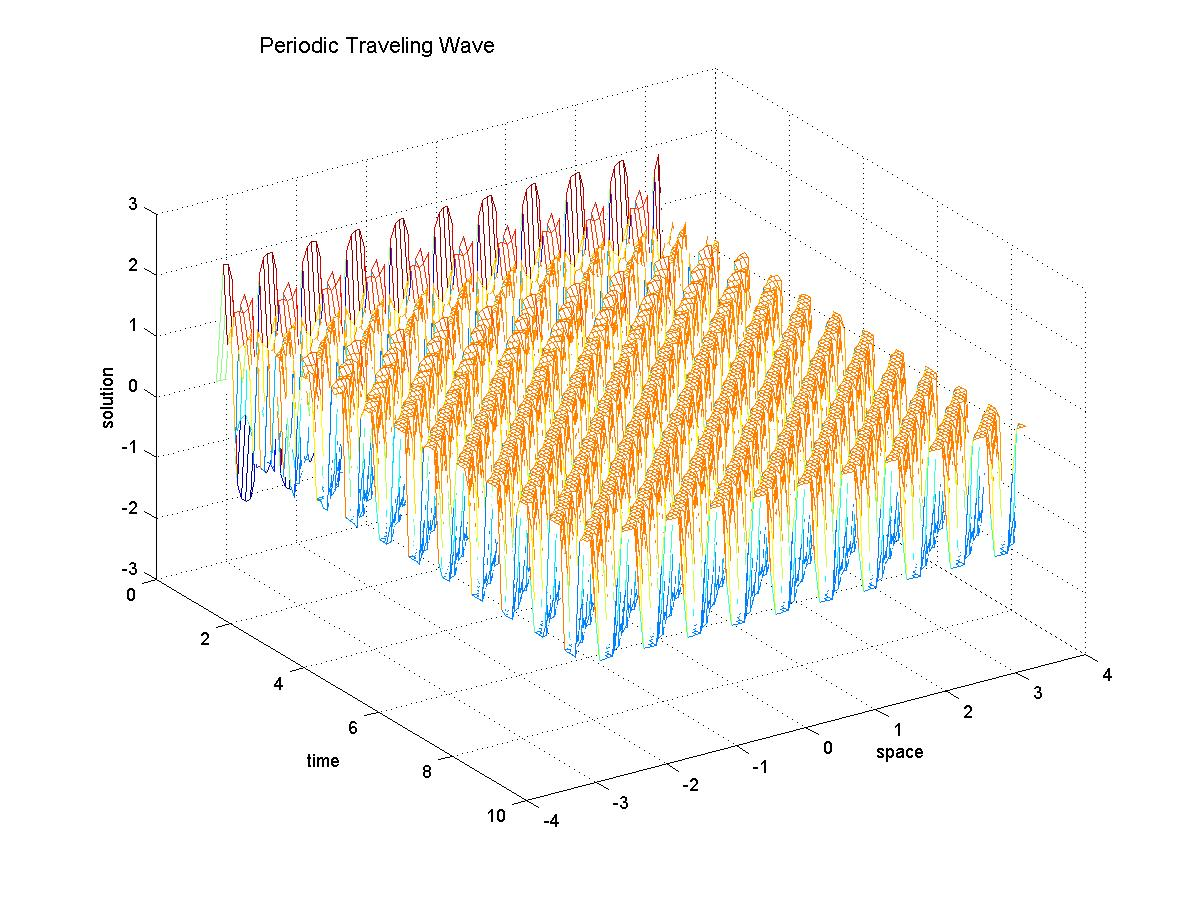Figure 3.1,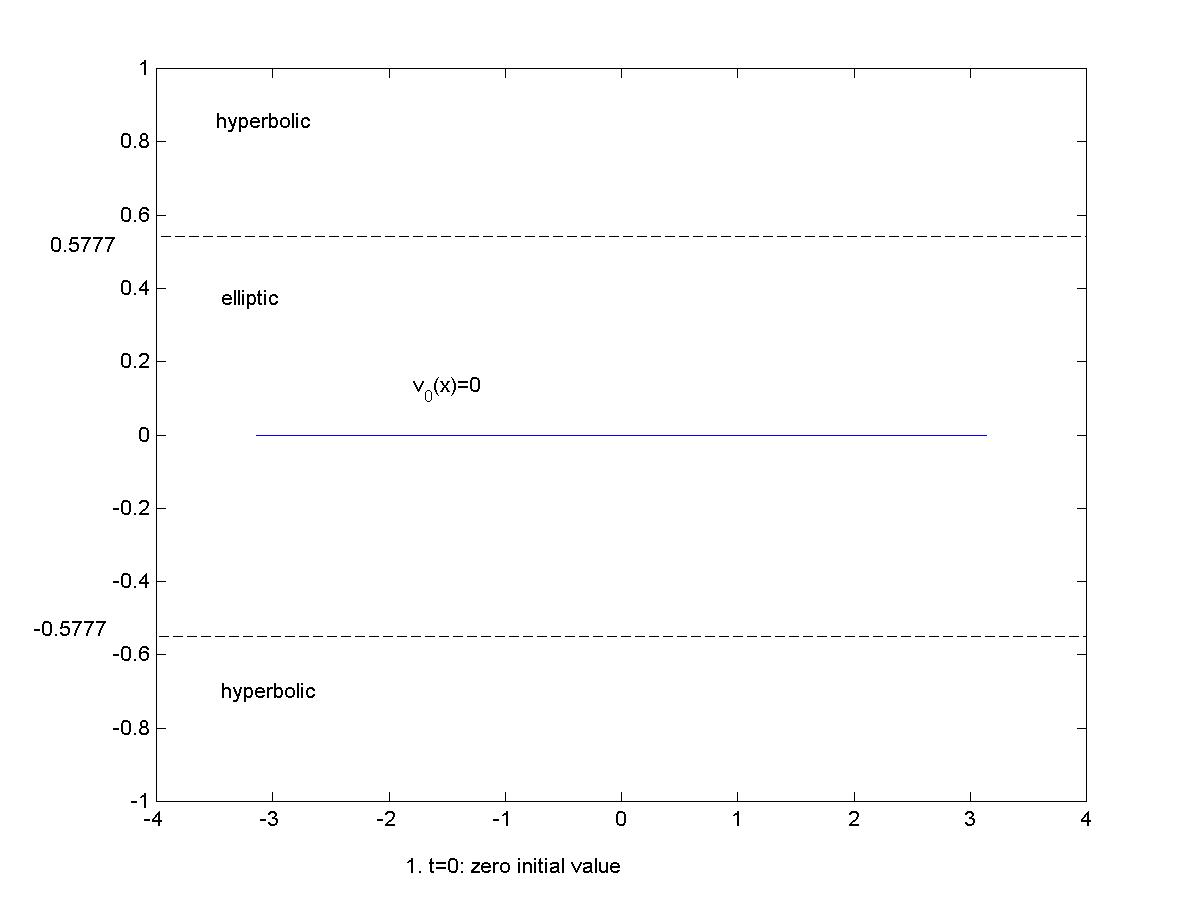Figure 3.2,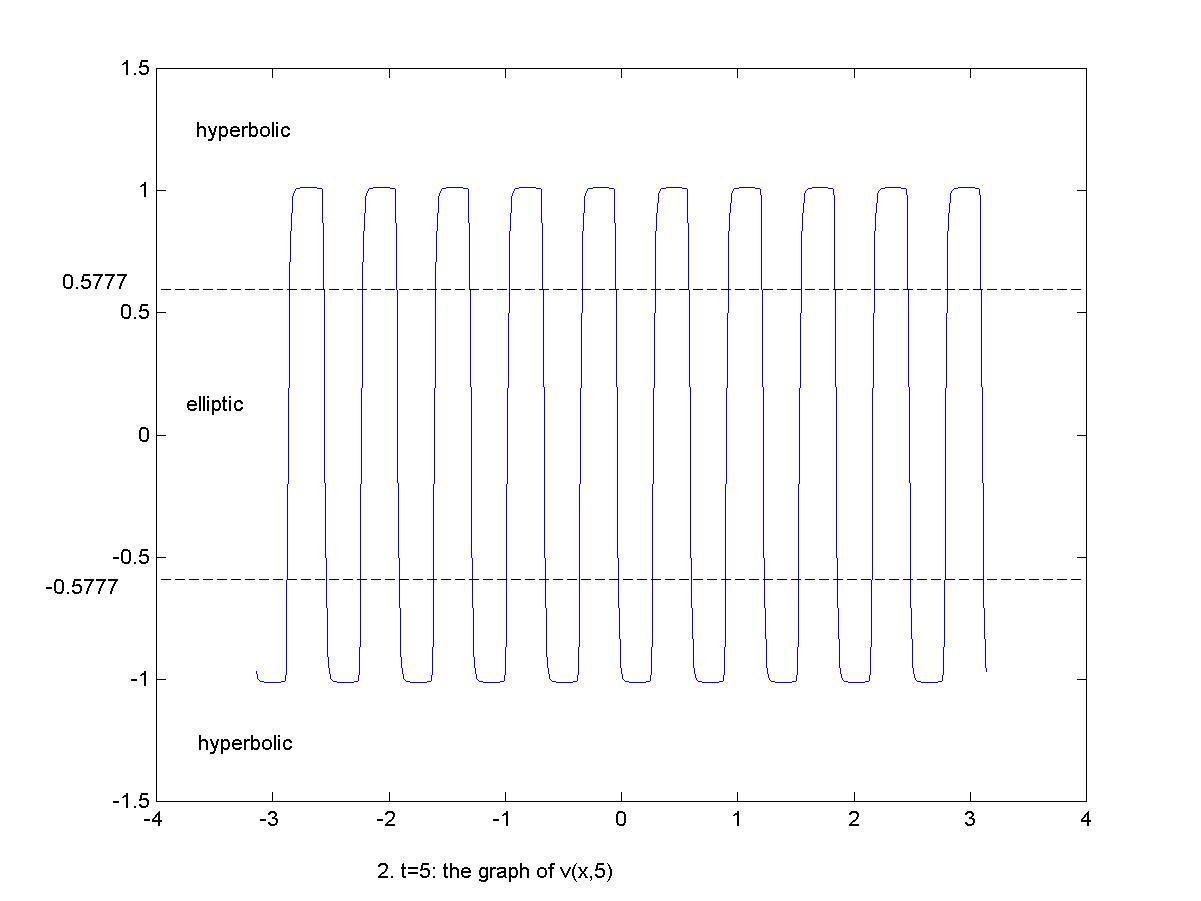Figure 3.3,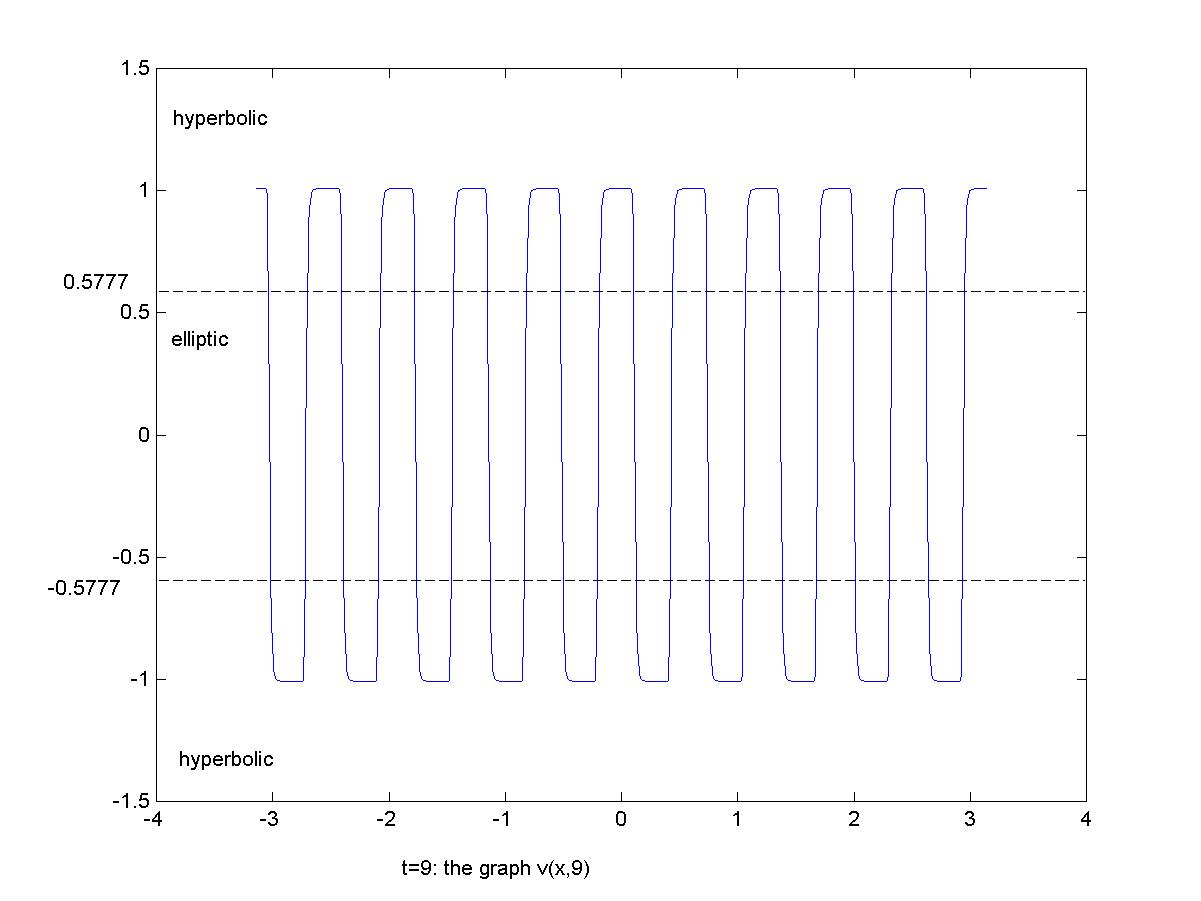Figure 3.4,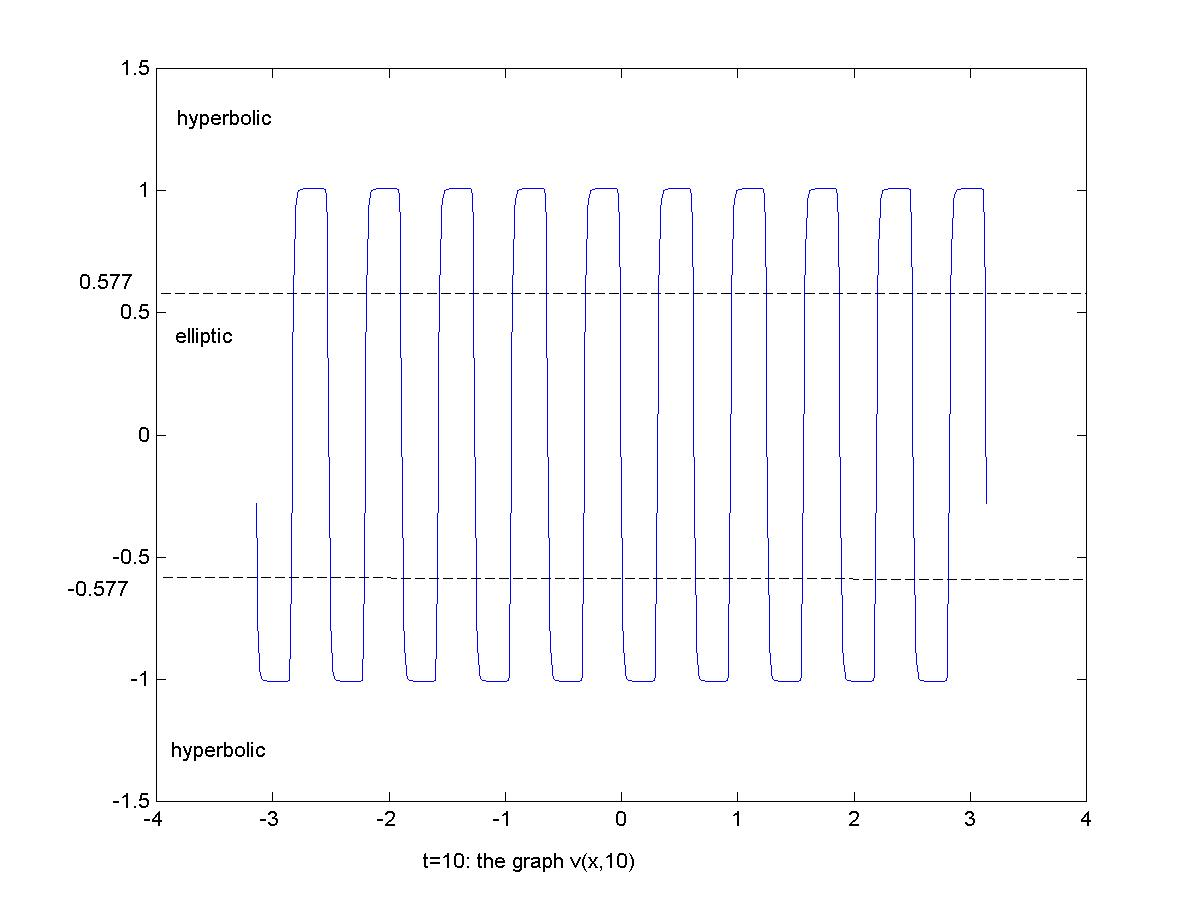Figure 3.5.